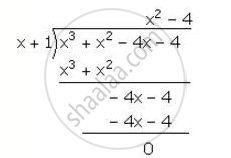Share

Using the Remainder Theorem, Factorise Each of the Following Completely X3 + X2 – 4x – 4 - ICSE Class 10 - Mathematics

ConceptFactorising a Polynomial Completely After Obtaining One Factor by Factor Theorem

Question

Using the Remainder Theorem, factorise each of the following completely

x3 + x2 – 4x – 4

Solution

f(x)=x^3+x^2-4x-4

for  x=1,

f(x)=f(-1)=(-1)^3+(-1)^2-4(-1)-4

=-1+1+4-4=0

"Hence, (x+1) is a factor of f (x)"∴ x^3+x^2-4x-4=(x+1)(x^2-4)

= (x+1) (x+2) (x-2)

Is there an error in this question or solution?

APPEARS IN

Solution Using the Remainder Theorem, Factorise Each of the Following Completely X3 + X2 – 4x – 4 Concept: Factorising a Polynomial Completely After Obtaining One Factor by Factor Theorem.
S